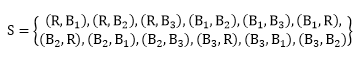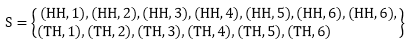×#### Thank you for registering.

One of our academic counsellors will contact you within 1 working day.

Click to Chat

1800-1023-196

+91-120-4616500

CART 0

• 0

MY CART (5)

Use Coupon: CART20 and get 20% off on all online Study Material

ITEM
DETAILS
MRP
DISCOUNT
FINAL PRICE
Total Price: Rs.

There are no items in this cart.
Continue Shopping• Complete JEE Main/Advanced Course and Test Series
• OFFERED PRICE: Rs. 15,900
• View Details

```Chapter 33: Probability – Exercise 33.1

Probability – Exercise 33.1 – Q.1

Since one coin is tossed, so there are two possibility either head turned up or tail.

SO, the sample space will be

S = {H,T}

Where, H - if head turned up.

T - if tail turned up.

Probability – Exercise 33.1 – Q.2

Since two coins are tossed, so the possibilities are either both coin shows head, or tail, or one shows head and other shows tail or vice-versa.

T represent tail

Thus, the sample space is given by,

S = {HT, TH, HH, TT}

Probability – Exercise 33.1 – Q.3

Since three coins are tossed. So, we have these possibilities.

(ii) All coins shows tail.

(iii) First two coins shows head and last coin shows tail.

(iv) First and third coins shows, head and second coin shows tail.

(v) Last two coins shows head and first coin shows tail.

(vi) First coin shows head and last two coins shows tail.

(vii) First and third coin shows tail and second coin shows head.

(viii) Third coin shows head and first two coins shows tail.

So, the number of element in sample space = 23= 8

Thus, the sample will be,

S = {HHH, TTT, HHT, HTH, THH, HTT, THT, TTH}

Probability – Exercise 33.1 – Q.4

Since four coins are tossed, so the possibilities are either

HHHH or TTTT or HHHT or HHTH or HTHH or THHH or HHTT or HTTH or HTHT or THHT or THTH or TTHH or HTTT or THTT or TTHT or TTTH

It means nos of elements in sample space = 24= 16

S = {(HHHH, TTTT, HHHT, HHTH, HTHH, THHH, HHTT, HTTH, HTHT, THHT, THTH, TTHH, HTTT, THTT, TTHT, TTTH)}

Probability – Exercise 33.1 – Q.5

In a dice there are six faces with numbers 1, 2, 3, 4, 5, 6

So, when two dice are thrown, then we have two faces of dice (one of each) show any two combination of numbers from 1, 2, 3, 4, 5, 6

Thus, the nos of element in sample space = 62 = 36Probability – Exercise 33.1 – Q.6

Since three dice are thrown together, so each of the three dice will show one face with number 1, 2, 3, 4, 5 or 6.

So, the total number of elementary events associated is 6 × 6 × 6 = 216.

Probability – Exercise 33.1 – Q.7

∵ When a coin is tossed, either tail or head will turn up, where as when a dice is thrown, we have one face with either of 1, 2, 3, 4, 5 or 6.

So, the total number of elementary events associated with this experiment is 2 x6 • 12 and the sample space will be

S = {(H, 1), (H, 2), (H, 3), (H, 4), (H, 5), (H, 6), (T, 1), (T, 2), (T, 3), (T, 4), (T, 5), (T, 5)}

Probability – Exercise 33.1 – Q.8

When a coin is tossed either head or tail will turn up. And, when head turns up then a dice is rolled otherwise not.

So, the total number of elementary events associated with this experiment is 1 + 6 × 1 = 7

Thus, the sample space will be

S = {T, (H, 1), (H, 2), (H, 3), (H, 4), (H, 5), (H, 6)}

Probability – Exercise 33.1 – Q.9

When a coin is tossed two times, then we have the following possibilities

HH, TT, TH and TT

Now, according to the question, when we have tail in 2nd throw, then a dice is thrown.

So, the total number of elementary events associated with this experiment are

2 + 2 × 614

and the sample space will beProbability – Exercise 33.1 – Q.10

In this experiment, a coin is tossed and if the outcome is tail then a die is tossed once.

Otherwise, the coin is tossed again.

The possible outcome for coin is either head or tail.

The possible outcome for die is 1, 2, 3, 4, 5, 6.

If the outcome for the coin is tail then sample space is S1 = {(T, 1), (T, 2), (T, 3), (T, 4), (T, 5), (T, 6)}

If the outcome is head then the sample space is S2 = {(H, H), (H, T)}

Therefore the required sample space is S = {(T, 1), (T, 2), (T, 3), (T, 4), (T, 5), (T, 6), (H, H), (H, T)}

Probability – Exercise 33.1 – Q.11

∵ A coin is tossed, then we have either heads (H) or tails (T).

If tail turned up, then a ball is drawn from a box which has 2 red and 3 black balls.

So, S1 = {(T, R1), (T, R2), (T, B1), (T, B2), (T, B3)}

If head turned up, then die is rolled

So, S2 = {(H, 1), (H, 2), (H, 3), (H, 4), (H, 5), (H, 6)}

Thus, the elementary events associated with this experiment is

S = {S1 ∪S2}

= {(T, R1), (T, R2), (T, B1), (T, B2), (T, B3), (H, 1), (H, 2), (H, 3), (H,4), (H, 5), (H, 6)}

Probability – Exercise 33.1 – Q.12

In this experiment, a coin is tossed and if the outcome is tail the experiment is over.

Otherwise, the coin is tossed again.

In the second toss also if the outcome is tail the experiment is over, otherwise tossed again.

In the third toss, if the outcome is tail, the experiment is over, otherwise tossed again.

This process continues indefinitely.

Hence, the sample spas S associated to this random experiment is

S = {T, HT, HHT, HHHT, HHHHT,...}

Probability – Exercise 33.1 – Q.13

In a box 1 Red ball 3 Black ball

Since two balls are drawn without replacement then the elementary event associated with this experiment isProbability – Exercise 33.1 – Q.14

Since a pair of dice is rolled, so total number of elementary events = 62 = 36

Again, if the doublet is outcomes i.e., we have either (1, 1), (2, 2), (3, 3), (4, 4), (5, 5), (6, 6) then a coin is tossed, then we have H or T.

∴ Total number of elementary events = 6 × 2 = 12

Thus, the total number of elementary events = 30 + 12 = 42

Note: The doublet (1, 1), (2, 2), (3, 3), (4, 4), (5, 5), (6, 6) was also included in 36. So we look 30 in final conclusion.

Probability – Exercise 33.1 – Q.15

A coin is tossed twice. So, the elementary events are

S1 = {HH, HT, TH, TT}

Now,

if the second drawn results is head, then a die is rolled then the elementary events is rolled then the elementary event isThus, sample space associated with this experiment is S = S1 ∪ S2Probability – Exercise 33.1 – Q.16

Bag 4 red balls (identical)

3 black ball (identical)

∵A ball is drawn in first attempt, so elementary events is

S1 = {R, B}

Now, the ball will put into the bag and draw are again

S1 = (R, B)

Thus, the sample space associated is

S = S1S2 = (RR, RB, BR, 88}

Probability – Exercise 33.1 – Q.17

In a random sampling, three items are selected so it could be any of the following:

a) All defective or

b) All non-defective or

c) Combination of defective and non defective.

Sample space associated with this experiment is

S = {DDD, NDN, DND, DNN, NDD, DDN, NND, NNN}

Probability – Exercise 33.1 – Q.18

Since a family has two children

i) Then the sample space may be

S = {(B1, B2), (B1 G2), (G1, B2) (G1+ G2)}

when subscript 1 and 2 represent elder and younger children.

ii) Since the family has two children so, the following possibility of boys in the family

i) No boys only girls

ii) One boy and one girl

iii) Two boys only

∴ S = {0, 1, 2}

S = {0, 1, 2}
```### Course Features

• 728 Video Lectures
• Revision Notes
• Previous Year Papers
• Mind Map
• Study Planner
• NCERT Solutions
• Discussion Forum
• Test paper with Video Solution